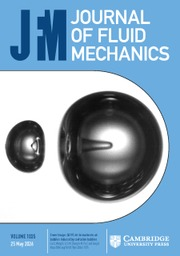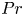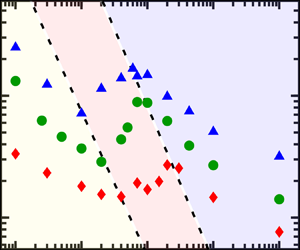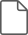Home
Hostname: page-component-7f7b94f6bd-w6m4b Total loading time: 0.403 Render date: 2022-06-29T08:13:44.621Z Has data issue: true Feature Flags: { "shouldUseShareProductTool": true, "shouldUseHypothesis": true, "isUnsiloEnabled": true, "useRatesEcommerce": false, "useNewApi": true } hasContentIssue trueJournal of Fluid Mechanics

# The$Pr$-dependence of the critical roughness height in two-dimensional turbulent Rayleigh–Bénard convection

Published online by Cambridge University Press:  01 February 2021

*

## AbstractWe carry out direct numerical simulations of turbulent Rayleigh–Bénard convection in a square box with rough conducting plates over the Rayleigh number range$10^7\leqslant Ra\leqslant 10^9$and the Prandtl number range$0.01\leqslant Pr\leqslant 100$. In Zhang et al. (J. Fluid Mech., vol. 836, 2018, R2), it was reported that while the measured Nusselt number$Nu$is enhanced at large roughness height$h$, the global heat transport is reduced at small$h$. The division between the two regimes yields a critical roughness height$h_c$, and we now focus on the effects of the Prandtl number ($Pr$) on$h_c$. Based on the variations of$h_c$, we identify three regimes for$h_c(Pr)$. For low$Pr$, thermal boundary layers become thinner with increasing$Pr$. This makes the boundary layers easier to be disrupted by rough elements, leading to the decrease of$h_c$with increasing$Pr$. For moderate$Pr$, the corner-flow rolls become much more pronounced and suppress the global heat transport via the competition between the corner-flow rolls and the large-scale circulation (LSC). As a consequence,$h_c$ increases with increasing$Pr$due to the intensification of the corner–LSC competition. For high$Pr$, the convective flow transitions to the plume-controlled regime. As the rough elements trigger much stronger and more frequent plume emissions,$h_c$again decreases with increasing$Pr$.

## JFM classification

Type
JFM Papers
Information
Journal of Fluid Mechanics , 25 March 2021 , A52

## Access options

Get access to the full version of this content by using one of the access options below. (Log in options will check for institutional or personal access. Content may require purchase if you do not have access.)

## References

Ahlers, G., Grossmann, S. & Lohse, D. 2009 Heat transfer and large scale dynamics in turbulent Rayleigh–Bénard convection. Rev. Mod. Phys. 81, 503537.CrossRefGoogle Scholar
Bao, Y., Chen, J., Liu, B.-F., She, Z.-S., Zhang, J. & Zhou, Q. 2015 Enhanced heat transport in partitioned thermal convection. J. Fluid Mech. 784, R5.CrossRefGoogle Scholar
Breuer, M., Wessling, S., Schmalzl, J. & Hansen, U. 2004 Effect of inertia in Rayleigh–Bénard convection. Phys. Rev. E 69, 026302.CrossRefGoogle ScholarPubMed
Chandra, M. & Verma, M.K. 2013 Flow reversals in turbulent convection via vortex reconnections. Phys. Rev. Lett. 110, 114503.CrossRefGoogle ScholarPubMed
Chillà, F. & Schumacher, J. 2012 New perspectives in turbulent Rayleigh–Bénard convection. Eur. Phys. J. E 35, 58.CrossRefGoogle ScholarPubMed
Ciliberto, S. & Laroche, C. 1999 Random roughness of boundary increases the turbulent convection scaling exponent. Phys. Rev. Lett. 82, 39984001.CrossRefGoogle Scholar
Du, Y.-B. & Tong, P. 1998 Enhanced heat transport in turbulent convection over a rough surface. Phys. Rev. Lett. 81, 987990.CrossRefGoogle Scholar
Du, Y.-B. & Tong, P. 2000 Turbulent thermal convection in a cell with ordered rough boundaries. J. Fluid Mech. 407, 5784.CrossRefGoogle Scholar
Dubrulle, B. 2002 Scaling in large Prandtl number turbulent thermal convection. Eur. Phys. J. B 28, 361367.CrossRefGoogle Scholar
Emran, M.S. & Shishkina, O. 2020 Natural convection in cylindrical containers with isothermal ring-shaped obstacles. J. Fluid Mech. 882, A3.CrossRefGoogle Scholar
Foroozani, N., Niemela, J.J., Armenio, V. & Sreenivasan, K.R. 2019 Turbulent convection and large scale circulation in a cube with rough horizontal surfaces. Phys. Rev. E 99, 033116.CrossRefGoogle Scholar
Frick, P., Khalilov, R., Kolesnichenko, I., Mamykin, A., Pakholkov, V., Pavlinov, A. & Rogozhkin, S. 2015 Turbulent convective heat transfer in a long cylinder with liquid sodium. Europhys. Lett. 14002, 16.Google Scholar
Grossmann, S. & Lohse, D. 2004 Fluctuations in turbulent Rayleigh–Bénard convection: the role of plumes. Phys. Fluids 16, 44624472.CrossRefGoogle Scholar
Grossmann, S. & Lohse, D. 2008 Thermal convection in small Prandtl number liquids: strong but ineffective. AIP Conf. Proc. 1076, 68.CrossRefGoogle Scholar
Grossmann, S. & Lohse, D. 2011 Multiple scaling in the ultimate regime of thermal convection. Phys. Fluids 23, 045108.CrossRefGoogle Scholar
He, X.-Z., Funfschilling, D., Nobach, H., Bodenschatz, E. & Ahlers, G. 2012 Transition to the ultimate state of turbulent Rayleigh–Bénard convection. Phys. Rev. Lett. 108, 024502.CrossRefGoogle ScholarPubMed
Horanyi, S., Krebs, L. & Müller, U. 1999 Turbulent Rayleigh–Bénard convection in low-Prandtl number. Intl J. Heat Mass Transfer 42, 39834003.CrossRefGoogle Scholar
Howard, L.N. 1963 Heat transport by turbulent convection. J. Fluid Mech. 17, 405432.CrossRefGoogle Scholar
Huang, Y.-X. & Zhou, Q. 2013 Counter-gradient heat transport in two-dimensional turbulent Rayleigh–Bénard convection. J. Fluid Mech. 737, R3.CrossRefGoogle Scholar
Jiang, H., Zhu, X., Mathai, V., Verzicco, R., Lohse, D. & Sun, C. 2018 Controlling heat transport and flow structures in thermal turbulence using ratchet surfaces. Phys. Rev. Lett. 120, 044501.CrossRefGoogle ScholarPubMed
Kaczorowski, M. & Xia, K.-Q. 2013 Turbulent flow in the bulk of Rayleigh–Bénard convection: small-scale properties in a cubic cell. J. Fluid Mech. 722, 596617.CrossRefGoogle Scholar
Kraichnan, R.H. 1962 Turbulent thermal convection at arbritrary Prandtl number. Phys. Fluids 5, 13741389.CrossRefGoogle Scholar
Lepot, S., Aumaître, S. & Gallet, B. 2018 Radiative heating achieves the ultimate regime of thermal convection. Proc. Natl Acad. Sci. USA 115, 89378941.CrossRefGoogle ScholarPubMed
Liot, O., Ehlinger, Q., Rusaouen, E., Coudarchet, T., Salort, J. & Chillà, F. 2017 Velocity fluctuations and boundary layer structure in a rough Rayleigh–Bénard cell filled with water. Phys. Rev. Fluids 2, 044605.CrossRefGoogle Scholar
Lohse, D. & Xia, K.-Q. 2010 Small-scale properties of turbulent Rayleigh–Bénard convection. Annu. Rev. Fluid Mech. 42, 335–64.CrossRefGoogle Scholar
MacDonald, M., Hutchins, N., Lohse, D. & Chung, D. 2019 Heat transfer in rough-wall turbulent thermal convection in the ultimate regime. Phys. Rev. Fluids 4, 071501(R).CrossRefGoogle Scholar
Malkus, M.V.R. 1954 The heat transport and spectrum of thermal turbulence. Proc. R. Soc. Lond. A 225, 196212.Google Scholar
Pandey, A. & Verma, M.K. 2016 Scaling of large-scale quantities in Rayleigh–Bénard convection. Phys. Fluids 28, 095105.CrossRefGoogle Scholar
van der Poel, E.P., Stevens, R.J.A.M. & Lohse, D. 2013 Comparison between two- and three-dimensional Rayleigh–Bénard convection. J. Fluid Mech. 736, 177194.CrossRefGoogle Scholar
Priestly, C.H.B. 1954 Convection from a large horizontal surface. Austral. J. Phys. 7, 176201.CrossRefGoogle Scholar
du Puits, R., Resagk, C. & Thess, A. 2010 Measurements of the instantaneous local heat flux in turbulent Rayleigh–Bénard convection. New J. Phys. 12, 075023.CrossRefGoogle Scholar
Qiu, X.-L., Xia, K.-Q. & Tong, P. 2005 Experimental study of velocity boundary layer near a rough conducting surface in turbulent natural convection. J. Turbul. 6, 30.CrossRefGoogle Scholar
Roche, R.-E., Castaing, B., Chabaud, B. & Hebral, B. 2001 Observation of the 1/2 power law in Rayleigh–Bénard convection. Phys. Rev. E 63, 045303(R).CrossRefGoogle Scholar
Roche, P.-E., Gauthier, F., Kaiser, R. & Salort, J. 2010 On the triggering of the Ultimate Regime of convection. New J. Phys. 12, 085014.CrossRefGoogle Scholar
Rusaouen, E., Liot, O., Castaing, B., Salort, J. & Chillà, F. 2018 Thermal transfer in Rayleigh–Bénard cell with smooth or rough boundaries. J. Fluid Mech. 837, 443460.CrossRefGoogle Scholar
Salort, J., Liot, O., Rusaouen, E., Seychelles, F., Tisserand, J.-C., Creyssels, M., Castaing, B. & Chillà, F. 2014 Thermal boundary layer near roughnesses in turbulent Rayleigh–Bénard convection: flow structure and multistability. Phys. Fluids 26, 015112.CrossRefGoogle Scholar
Scheel, J.D. & Schumacher, J. 2016 Global and local statistics in turbulent convection at low Prandtl numbers. J. Fluid Mech. 802, 147173.CrossRefGoogle Scholar
Shang, X.-D., Tong, P. & Xia, K.-Q. 2008 Scaling of the local convective heat flux in turbulent Rayleigh–Bénard convection. Phys. Rev. Lett. 100, 244503.CrossRefGoogle ScholarPubMed
Shishkina, O., Horn, S., Wagner, S. & Ching, E.S.C. 2015 Thermal boundary layer equation for turbulent Rayleigh–Bénard convection. Phys. Rev. Lett. 114, 114302.CrossRefGoogle ScholarPubMed
Shishkina, O. & Wagner, C. 2011 Modelling the influence of wall roughness on heat transfer in thermal convection. J. Fluid Mech. 686, 568582.CrossRefGoogle Scholar
Silano, G., Sreenivasan, K.R. & Verzicco, R. 2010 Numerical simulations of Rayleigh–Bénard convection for Prandtl numbers between$10^{-1}$ and$10^4$ and Rayleigh numbers between$10^5$ and$10^9$. J. Fluid Mech. 662, 409446.CrossRefGoogle Scholar
Spiegel, E.A. 1971 Convection in stars. Annu. Rev. Astron. Astrophys. 9, 323352.CrossRefGoogle Scholar
Stevens, R.J.A.M., Lohse, D. & Verzicco, R. 2011 Prandtl and Rayleigh number dependence of heat transport in high Rayleigh number thermal convection. J. Fluid Mech. 688, 3143.CrossRefGoogle Scholar
Stringano, G., Pascazio, G. & Verzicco, R. 2006 Turbulent thermal convection over grooved plates. J. Fluid Mech. 557, 307336.CrossRefGoogle Scholar
Sugiyama, K., Calzavarini, E., Grossmann, S. & Lohse, D. 2009 Flow organization in two-dimensional non-Oberbeck-Boussinesq Rayleigh–Bénard convection in water. J. Fluid Mech. 637, 105135.CrossRefGoogle Scholar
Sugiyama, K., Ni, R., Stevens, R.J.A.M., Chan, T.-S., Zhou, S.-Q., Xi, H.-D., Sun, C., Grossmann, S., Xia, K.-Q. & Lohse, D. 2010 Flow reversals in thermally driven turbulence. Phys. Rev. Lett. 105, 034503.CrossRefGoogle ScholarPubMed
Sun, C. & Zhou, Q. 2014 Experimental techniques for turbulent Taylor–Couette flow and Rayleigh–Bénard convection. Nonlinearity 27, R89R121.CrossRefGoogle Scholar
Tisserand, J.-C., Creyssels, M., Gasteuil, Y., Pabiou, H., Gibert, M., Castaing, B. & Chillà, F. 2011 Comparison between rough and smooth plates within the same Rayleigh–Bénard cell. Phys. Fluids 23, 015105.CrossRefGoogle Scholar
Toppaladoddi, S., Succi, S. & Wettlaufer, J.S. 2015 Tailoring boundary geometry to optimize heat transport in turbulent convection. Europhys. Lett. 111, 44005.CrossRefGoogle Scholar
Toppaladoddi, S., Succi, S. & Wettlaufer, J.S. 2017 Roughness as a route to the ultimate regime of thermal convection. Phys. Rev. Lett. 118, 074503.CrossRefGoogle ScholarPubMed
Verzicco, R. & Camussi, R. 1999 Prandtl number effects in convective turbulence. J. Fluid Mech. 383, 5573.CrossRefGoogle Scholar
Wang, Z., Mathai, V. & Sun, C. 2019 Self-sustained biphasic catalytic particle turbulence. Nat. Commun. 10, 3333.CrossRefGoogle ScholarPubMed
Wang, B.-F., Zhou, Q. & Sun, C. 2020 Vibration-induced boundary-layer destabilization achieves massive heat-transport enhancement. Sci. Adv. 6, eaaz8239.CrossRefGoogle ScholarPubMed
Wei, P., Chan, T.-S., Ni, R., Zhao, X.-Z. & Xia, K.-Q. 2014 Heat transport properties of plates with smooth and rough surfaces in turbulent thermal convection. J. Fluid Mech. 740, 2846.CrossRefGoogle Scholar
Whitehead, J.P. & Doering, C.R. 2011 Ultimate state of two-dimensional Rayleigh–Bénard convection between free-slip fixed-temperature boundaries. Phys. Rev. Lett. 106, 244501.CrossRefGoogle ScholarPubMed
Xi, H.-D., Lam, S. & Xia, K.-Q. 2004 From laminar plumes to organized flows: the onset of large-scale circulation in turbulent thermal convection. J. Fluid Mech. 503, 4756.CrossRefGoogle Scholar
Xia, K.-Q., Lam, S. & Zhou, S.-Q. 2002 Heat-flux measurement in high-Prandtl-number turbulent Rayleigh–Bénard convection. Phys. Rev. Lett. 88, 064501.CrossRefGoogle ScholarPubMed
Xia, K.-Q., Xin, Y.-B. & Tong, P. 1995 Dual-beam incoherent cross-correlation spectroscopy. J. Opt. Soc. Am. A 12, 1571.CrossRefGoogle Scholar
Xie, Y.-C. & Xia, K.-Q. 2017 Turbulent thermal convection over rough plates with varying roughness geometries. J. Fluid Mech. 825, 573599.CrossRefGoogle Scholar
Xin, Y.-B., Xia, K.-Q. & Tong, P. 1996 Measured velocity boundary layers in turbulent convection. Phys. Rev. Lett. 77, 12661269.CrossRefGoogle ScholarPubMed
Xu, A., Shi, L. & Xi, H.-D. 2019 Statistics of temperature and thermal energy dissipation rate in low-Prandtl number turbulent thermal convection. Phys. Fluids 31, 125101.Google Scholar
Xu, B.-L., Wang, Q., Wan, Z.-H., Yan, R. & Sun, D.-J. 2018 Heat transport enhancement and scaling law transition in two-dimensional Rayleigh–Bénard convection with rectangular-type roughness. Intl J. Heat Mass Transfer 121, 872883.CrossRefGoogle Scholar
Zhang, Y.-Z., Sun, C., Bao, Y. & Zhou, Q. 2018 How surface roughness reduces heat transport for small roughness heights in turbulent Rayleigh–Bénard convection. J. Fluid Mech. 836, R2.CrossRefGoogle Scholar
Zhang, Y.-Z., Xia, S.-N., Dong, Y.-H., Wang, B.-F. & Zhou, Q. 2019 An efficient parallel algorithm for dns of buoyancy-driven turbulent flows. J. Hydrodyn. 31 (6), 11591169.CrossRefGoogle Scholar
Zhang, Y., Zhou, Q. & Sun, C. 2017 Statistics of kinetic and thermal energy dissipation rates in two-dimensional turbulent Rayleigh–Bénard convection. J. Fluid Mech. 814, 165184.CrossRefGoogle Scholar
Zhou, Q. & Xia, K.-Q. 2013 Thermal boundary layer structure in turbulent Rayleigh–Bénard convection in a rectangular cell. J. Fluid Mech. 721, 199224.CrossRefGoogle Scholar
Zhu, X., Jiang, L.-F., Zhou, Q. & Sun, C. 2019 a Turbulent Rayleigh–Bénard convection in an annular cell. J. Fluid Mech. 869, R5.CrossRefGoogle Scholar
Zhu, X.-J., Stevens, R.J.A.M., Shishkina, O., Verzicco, R. & Lohse, D. 2019 b$Nu\sim Ra^{1/2}$ scaling enabled by multiscale wall roughness in Rayleigh–Bénard turbulence. J. Fluid Mech. 869, R4.CrossRefGoogle Scholar
Zhu, X.-J., Stevens, R.J.A.M., Verzicco, R. & Lohse, D. 2017 Roughness-facilitated local 1/2 scaling does not imply the onset of the ultimate regime of thermal convection. Phys. Rev. Lett. 119, 154501.CrossRefGoogle Scholar
Zwirner, L. & Shishkina, O. 2018 Confined inclined thermal convection in low-Prandtl-number fluids. J. Fluid Mech. 850, 9841008.CrossRefGoogle Scholar### Yang et al. supplementary material

Captions for movies 1-4

File 13 KB
12
Cited by

# Save article to Kindle

Note you can select to save to either the @free.kindle.com or @kindle.com variations. ‘@free.kindle.com’ emails are free but can only be saved to your device when it is connected to wi-fi. ‘@kindle.com’ emails can be delivered even when you are not connected to wi-fi, but note that service fees apply.

Find out more about the Kindle Personal Document Service.

The$Pr$-dependence of the critical roughness height in two-dimensional turbulent Rayleigh–Bénard convection Available formats × # Save article to Dropbox To save this article to your Dropbox account, please select one or more formats and confirm that you agree to abide by our usage policies. If this is the first time you used this feature, you will be asked to authorise Cambridge Core to connect with your Dropbox account. Find out more about saving content to Dropbox. The$Pr$-dependence of the critical roughness height in two-dimensional turbulent Rayleigh–Bénard convection
Available formats
×

# Save article to Google Drive

To save this article to your Google Drive account, please select one or more formats and confirm that you agree to abide by our usage policies. If this is the first time you used this feature, you will be asked to authorise Cambridge Core to connect with your Google Drive account. Find out more about saving content to Google Drive.

The$Pr\$-dependence of the critical roughness height in two-dimensional turbulent Rayleigh–Bénard convection
Available formats
×
×